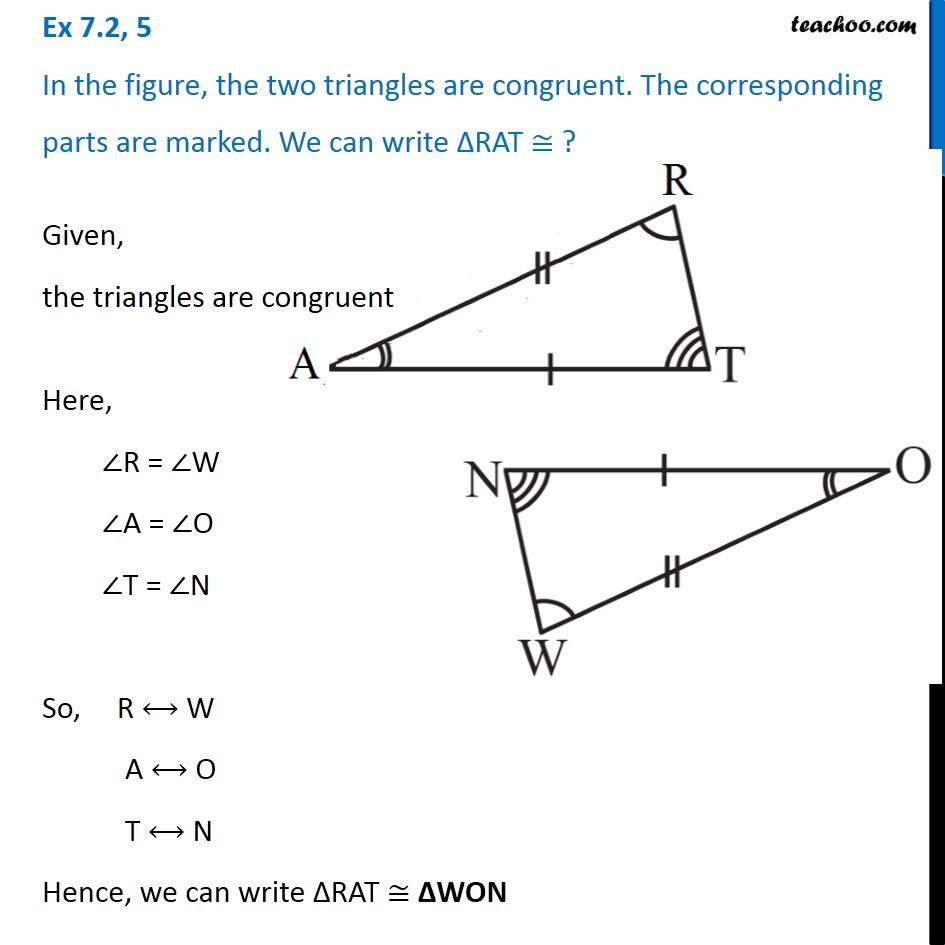Ex 7.2

Chapter 7 Class 7 Congruence of Triangles
Serial order wise### Transcript

Ex 7.2, 5 In the figure, the two triangles are congruent. The corresponding parts are marked. We can write ∆RAT ≅ ? Given, the triangles are congruent Here, ∠R = ∠W ∠A = ∠O ∠T = ∠N So, R ⟷ W A ⟷ O T ⟷ N Hence, we can write ∆RAT ≅ ∆WON